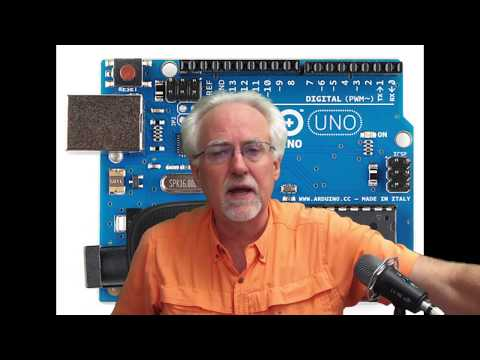# Arduino Tutorial 47: Binary and Hexadecimal Bit FlipperIn this lesson we explore how to create a Binary or Hexadecimal Bit Flipper. From our earlier lessons you see we can visually represent Hexadecimal or Binary numbers with a series of LED, with an on LED representing a “1” and an off LED representing a “0”. In programming and circuit applications, it is sometimes useful to “flip” or invert the bits. For an 8 bit number, one could do this in a program with 255 IF statements, but there is a simpler way. If you think about it, you can get the flippedByte by simple subtracting the byte from 0xFF, or 0b11111111. If you try some test cases, you can see that this will always work.

Simply stated, flippedByte=0xFF-Byte,

or if you prefer thinking in binary,

flippedByte=0b11111111-Byte

This is the circuit we are using to drive the 8 LED with the 74HX595 chip, and all this was explained in Tutorial 42.This is the schematic we use in this example to control 8 LEDs from the 74HC595 chip.

This is the code which we developed in the video above.

In all these lessons we are using the Arduino Super Starter Kit, which you can pick up HERE.